# TSA Bispectrum VI

LabVIEW 2014 Advanced Signal Processing Toolkit Help

Edition Date: June 2014

Part Number: 372656C-01

»View Product InfoDownload Help (Windows Only)

Owning Palette: Correlation and Spectral Analysis VIs

Computes the single-sided bispectrum of a univariate time series using the fast Fourier transform (FFT) or the autoregressive (AR) model based method. The bispectrum is a type of third-order spectrum, which is related to the third moment (skewness) of a time series. The resulting bispectrum can detect the asymmetric nonlinearities in the input time series. Wire data to the Xt input to determine the polymorphic instance to use or manually select the instance.

Use the pull-down menu to select an instance of this VI.

 Select an instance TSA Bispectrum (Waveform)TSA Bispectrum (Array)

## TSA Bispectrum (Waveform)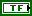initialize? specifies whether the input block of data is the first block. The default is FALSE, which means the input block of data is not the first block. When the input block of data is not the first block, this VI initializes the internal state of the block of data to the final state by using the previous call to this VI instance. When initialize? is TRUE, the input block of data is the first block and this VI initializes the internal state of the block of data to zero and processes one block of data.

To process consecutive blocks of data, set initialize? to TRUE for the first block and FALSE for all other blocks of data.frequency bins specifies the number of frequency bins for which this VI computes the bispectrum. The resulting bispectrum S(f,f) is a square 2D array with the size (frequency bins/2+1). The default is –1, which means this VI calculates an appropriate value for the frequency bins. Refer to the Details section for information about how this VI calculates the frequency bins value.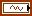Xt specifies the univariate time series.method specifies to compute the bispectrum with the fast Fourier transform (FFT) based or autoregressive (AR) model based method. The default is FFT. Refer to the Details section for more information about each method.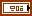window info specifies the information of the sliding window that divides the time series into subsequences.window specifies the time-domain window applied to the time series. Options include None (default), Hanning, Hamming, Blackman-Harris, Exact Blackman, Blackman, Flat Top, 4 Term B-Harris, 7 Term B-Harris, and Low Sidelobe.length specifies the length of the window. A large window generates a power spectral density (PSD) with small bias but results in a coarse PSD plot. A small window generates a smooth PSD plot but leads to large bias. The default is –1, which indicates that the window length equals the length of the input time series.overlap specifies the overlap, in percentage, of the moving window that this VI applies to the time series. This parameter determines how much data this VI reuses for the signal space matrix. A large overlap reduces the variance of the resulting power spectrum but increases computation time. The default is 50, which specifies that the overlap is half of the window length.error in describes error conditions that occur before this node runs. This input provides standard error in functionality.dB on? specifies whether this VI returns the S(f,f) in decibels or in a linear scale. If dB on? is TRUE, this VI returns the S(f,f) in decibels. If dB on? is FALSE, this VI returns the S(f,f) in a linear scale. The default is TRUE.averaging parameters specifies how this VI computes the averaging. The specifications of the parameters include the type of averaging, the type of weighting, and the number of averages.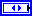averaging mode specifies the averaging mode.

 0 No averaging (Default) 1 Vector averaging 2 RMS averaging 3 Peak holdweighting mode specifies the weighting mode for RMS and vector averaging.

 0 Linear 1 Exponential (Default)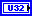number of averages specifies the number of averages used for RMS and vector averaging. If weighting mode is exponential, the averaging process is continuous. If weighting mode is linear, the averaging process stops after this VI computes the selected number of averages.AR setting specifies the settings for the autoregressive (AR) model. This option is valid only when the method is AR Model.AR method specifies the method this VI uses to estimate the autoregressive (AR) model.

 0 Forward-Backward (default)—Computes the AR coefficients by minimizing the least-square errors of the forward and backward predictions. 1 Least-Squares—Computes the AR coefficients by minimizing the least-square errors of the forward predictions. 2 Yule-Walker—Computes the AR coefficients by solving the Yule-Walker functions based on the forward predictions. 3 Burg-Lattice—Computes the AR coefficients using the Levinson-Durbin recursion based on the forward and backward predictions. The Levinson-Durbin recursion uses the arithmetic average. 4 Geometric-Lattice—Computes the AR coefficients using the Levinson-Durbin recursion based on the forward and backward predictions. The Levinson-Durbin recursion uses the geometric average.AR order specifies the order of the autoregressive (AR) model. The value of AR order must be greater than 0. The default is 4.S(f,f) returns the magnitude of the single-sided bispectrum S(f1, f2).freq bins returns the frequency bins at which this VI estimates the bispectrum.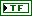averaging done? returns whether the averaging process is complete. averaging done? returns TRUE when the number of averages this VI completes is greater than or equal to the number of averages specified in averaging parameters. Otherwise, averaging done? returns FALSE. averaging done? is always TRUE if the selected averaging mode is No averaging.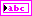unit returns the engineering unit of the PSD. You can specify an engineering unit for a time series by using the TSA Scale to EU VI.error out contains error information. This output provides standard error out functionality.

## TSA Bispectrum (Array)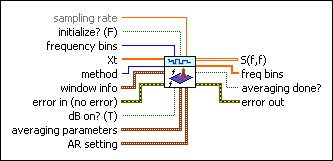sampling rate specifies the sampling rate, in hertz, of the univariate time series Xt. The default is 1.initialize? specifies whether the input block of data is the first block. The default is FALSE, which means the input block of data is not the first block. When the input block of data is not the first block, this VI initializes the internal state of the block of data to the final state by using the previous call to this VI instance. When initialize? is TRUE, the input block of data is the first block and this VI initializes the internal state of the block of data to zero and processes one block of data.

To process consecutive blocks of data, set initialize? to TRUE for the first block and FALSE for all other blocks of data.frequency bins specifies the number of frequency bins for which this VI computes the bispectrum. The resulting bispectrum S(f,f) is a square 2D array with the size (frequency bins/2+1). The default is –1, which means this VI calculates an appropriate value for the frequency bins. Refer to the Details section for information about how this VI calculates the frequency bins value.Xt specifies the univariate time series.method specifies to compute the bispectrum with the fast Fourier transform (FFT) based or autoregressive (AR) model based method. The default is FFT. Refer to the Details section for more information about each method.window info specifies the information of the sliding window that divides the time series into subsequences.window specifies the time-domain window applied to the time series. Options include None (default), Hanning, Hamming, Blackman-Harris, Exact Blackman, Blackman, Flat Top, 4 Term B-Harris, 7 Term B-Harris, and Low Sidelobe.length specifies the length of the window. A large window generates a power spectral density (PSD) with small bias but results in a coarse PSD plot. A small window generates a smooth PSD plot but leads to large bias. The default is –1, which indicates that the window length equals the length of the input time series.overlap specifies the overlap, in percentage, of the moving window that this VI applies to the time series. This parameter determines how much data this VI reuses for the signal space matrix. A large overlap reduces the variance of the resulting power spectrum but increases computation time. The default is 50, which specifies that the overlap is half of the window length.error in describes error conditions that occur before this node runs. This input provides standard error in functionality.dB on? specifies whether this VI returns the S(f,f) in decibels or in a linear scale. If dB on? is TRUE, this VI returns the S(f,f) in decibels. If dB on? is FALSE, this VI returns the S(f,f) in a linear scale. The default is TRUE.averaging parameters specifies how this VI computes the averaging. The specifications of the parameters include the type of averaging, the type of weighting, and the number of averages.averaging mode specifies the averaging mode.

 0 No averaging (Default) 1 Vector averaging 2 RMS averaging 3 Peak holdweighting mode specifies the weighting mode for RMS and vector averaging.

 0 Linear 1 Exponential (Default)number of averages specifies the number of averages used for RMS and vector averaging. If weighting mode is exponential, the averaging process is continuous. If weighting mode is linear, the averaging process stops after this VI computes the selected number of averages.AR setting specifies the settings for the autoregressive (AR) model. This option is valid only when the method is AR Model.AR method specifies the method this VI uses to estimate the autoregressive (AR) model.

 0 Forward-Backward (default)—Computes the AR coefficients by minimizing the least-square errors of the forward and backward predictions. 1 Least-Squares—Computes the AR coefficients by minimizing the least-square errors of the forward predictions. 2 Yule-Walker—Computes the AR coefficients by solving the Yule-Walker functions based on the forward predictions. 3 Burg-Lattice—Computes the AR coefficients using the Levinson-Durbin recursion based on the forward and backward predictions. The Levinson-Durbin recursion uses the arithmetic average. 4 Geometric-Lattice—Computes the AR coefficients using the Levinson-Durbin recursion based on the forward and backward predictions. The Levinson-Durbin recursion uses the geometric average.AR order specifies the order of the autoregressive (AR) model. The value of AR order must be greater than 0. The default is 4.S(f,f) returns the magnitude of the single-sided bispectrum S(f1, f2).freq bins returns the frequency bins at which this VI estimates the bispectrum.averaging done? returns whether the averaging process is complete. averaging done? returns TRUE when the number of averages this VI completes is greater than or equal to the number of averages specified in averaging parameters. Otherwise, averaging done? returns FALSE. averaging done? is always TRUE if the selected averaging mode is No averaging.error out contains error information. This output provides standard error out functionality.

## TSA Bispectrum Details

This VI computes the single-sided bispectrum of a univariate time series using the FFT based method according to the following equation:

S(f1, f2) = A[X(f1)X(f2)X*(f1+f2)]

A[X] denotes the averaging value of X. X(f) is the Fourier transform of the time series Xt according to the following equation.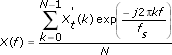where N is the number of frequency bins and fs is the sampling rate. Before computing the bispectrum, this VI adjusts the value of the window length, length, and the value of frequency bins according to the following table.

<=1024 –1 signal block size –1 signal block size
>1024 –1 1024 –1 1024
arbitrary arbitrary no change –1 length
arbitrary –1 signal block size <signal block size signal block size
arbitrary –1 signal block size >=signal block size no change
arbitrary arbitrary no change >=length no change
arbitrary arbitrary no change <length length

This VI computes the bispectrum of a univariate time series using the AR model based method according to the following equation:

S(f1, f2) = bA[X(f1)X(f2)X*(f1+f2)]

b is the third moment of estimated noise series of AR model.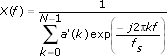s2 is the noise variance of the estimated AR model of the time series and a is an array that contains the coefficients of the AR model. a=[1, a1, a2, … ,an], where n is AR order. Before computing the bispectrum, this VI wraps a to an N-point series a'.

## Examples

Refer to the following VIs for examples of using the TSA Bispectrum VI:

• Bispectrum Analysis VI: labview\examples\Time Series Analysis\TSAGettingStarted
• Beam Crack Detection VI: labview\examples\Time Series Analysis\TSAApplications